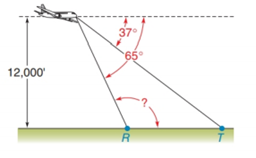Chapter 2.4, Problem 36EElementary Geometry For College St...

7th Edition
Alexander + 2 others
ISBN: 9781337614085

Solutions

Chapter
SectionElementary Geometry For College St...

7th Edition
Alexander + 2 others
ISBN: 9781337614085
Textbook Problem

An airplane has leveled off (is flying horizontally) at an altitude of 12, 000 feet. Its pilot can see each of two farmhouses at points R and T in front of the plane. With angle measures as indicated, find m ∠ RTo determine

To find:

The value of mR.

Explanation

Given:

The airplane is at an altitude of 12, 000 feet.

Figure (1)

Approach:

The sum of the interior angles on the same side of the transversal which cut by two parallel lines is equal to 180°.

Calculation:

R and 65° are two angles on the same side of the transversal as shown in figure (1).

Still sussing out bartleby?

Check out a sample textbook solution.

See a sample solution

The Solution to Your Study Problems

Bartleby provides explanations to thousands of textbook problems written by our experts, many with advanced degrees!

Get Started

The second partial derivative zyx of the function z=x2y+yex2 at the point (1,2).

Mathematical Applications for the Management, Life, and Social Sciences

Sketch the graphs of the equations in Exercises 512. xy=x2+1

Finite Mathematics and Applied Calculus (MindTap Course List)

If f(x)=x2xx1andg(x)=x is it true that f = g?

Single Variable Calculus: Early Transcendentals

Divide the following fractions and reduce to lowest terms. 22.

Contemporary Mathematics for Business & Consumers

True or False: f(x) = x2 is decreasing for 10 x 1.

Study Guide for Stewart's Single Variable Calculus: Early Transcendentals, 8th